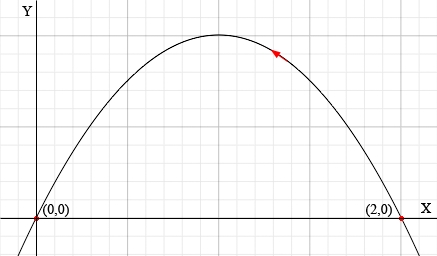SEARCH HOMEMath Central Quandaries & QueriesQuestion from stephanie, a student: Give parameterizations r(t)=x(t)i + y(t)j for the part of the parabola y=2x-x^2, from (2,0) to (0,0). Sketch the curve using arrows to show direction for increasing t. Essentially, i want to know how to determine the direction a particle is moving in for any curve, i have a vague idea using r'(t). Also, how do i parameterize? x=? and y=? Ans: x=2-t, y=2(2-t)-(2-t)^2Hi Stephanie,

You gave the answer as x = 2 - t, y = 2(2 - t) - (2 - t)2 but you missed an important part of the answer. You didn't give the domain of t and without it you don't know which part of the parabola you are parameterizing. You need to say

x = 2 - t, y = 2(2 - t) - (2 - t)2 for 0 ≤ t ≤ 2.

Now you can see that when t = 0, x = 2 and y = 0 and when t = 2, x = 0 and y = 0. Thus the parameterization is for the piece of the parabola from (2,0) to (0,0) but not only that, as t increases you are moving from (2,0) to (0,0).There are many ways to parameterize a curve and this is not the only answer to your problem. When I first read your problem I thought of the parameterization x = s and y = 2s - s2. In this case the point (2,0) comes from s = 2 and the point (0,0) comes from s = 0. Thus I get

x = s and y = 2s - s2 for 0 ≤ s ≤ 2.

With this parameterization as the parameter s increases you move along the curve from (0,0) to (2,0).

I hope this helps,
HarleyMath Central is supported by the University of Regina and The Pacific Institute for the Mathematical Sciences.